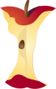Home > Grade Levels > High School Geometry >

Finding the Equation of a ParabolaAligned To Common Core Standard:

Expressing Properties - HSG-GPE.A.2(Click Here to Upgrade)

Homework Sheets

Find the equation when you are given the focus and directrix of a parabola.

Practice Worksheets

The practice sheets add one slight new skill to the mix.

Math Skill Quizzes

The quizzes also look for the vertex and axis of parabolas.

 Quiz 1 Quiz 2 Quiz 3